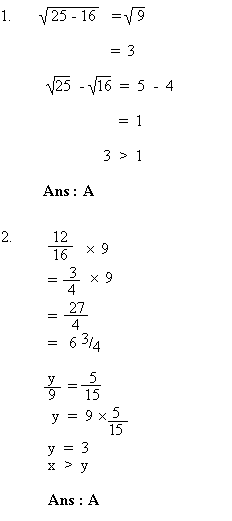Your browser does not support the IFRAME tag.Home MonkeyNotes Printable Notes Digital Library Study Guides Study Smart Parents Tips College Planning Test Prep Fun Zone Help / FAQ How to Cite New Title Request

 Section 4 : Mathematical Reasoning Part I : Quantitative Comparison QuestionsProduct of any number with zero is 0 Ans : C 4 - 5 < c - d < 10 - 7 -1 < c - d < 3 3 > c - d Ans : A Prime numbers are 2, 3, 5, 7, 11, 13, 17 and ... so on. b could be any of the above prime numbers. Given a = 7x - 2 where 7x is a multiple of 7. The relationship cannot be determined from the given data. Ans : D In the given right angled triangle, (hypotenuse)2 = The sum of the squares of the other 2 sides. (12 Ö 2 )2 = 122 + ?2 288 = 144 + ?2 \ ?2 = 144 \ ? = 12 Since this is an isosceles right triangle, a = 45 Ans : C Given t ¹0 then there are two possibilities: i) Either t > 0 or ii) t < 0. If t > 0, suppose t = 3. Then t2 + 2t = 8, and t2 - 2t = 0. In this case, LHS > RHS. If t < 0, suppose t = -1. Then t2 + 2t = -1, and t2 - 2t = 3 In this case, LHS < RHS. This means that from the given information we cannot determine the relationship between the two columns. Hence the choice is D. Ans : D 2h = 2 p r    r = 2h         2p    r = h / p 2 l = 2 r    r = l 8 l = 8 r 2h = 2 ´ r p 2h = 6.28 r 8 l > 2 h Ans : A Index PART IV Drill : Section 1 : Verbal Reasoning Section 2 : Mathematical Reasoning Section 3 : Verbal Reasoning Section 4 : Mathematical Reasoning Section 5 : Reading Comprehension Section 6 : Mathematical Reasoning Section 7 : Verbal Reasoning Answer Explanation To The Drill Section 1 : Verbal Reasoning Section 2 : Mathematical Reasoning Section 3 : Verbal Reasoning Section 4 : Mathematical Reasoning Section 5 : Reading Comprehension Section 6 : Mathematical Reasoning Section 7 : Verbal Reasoning Test 1
 Search: All Products Books Popular Music Classical Music Video DVD Toys & Games Electronics Software Tools & Hardware Outdoor Living Kitchen & Housewares Camera & Photo Cell Phones Keywords: